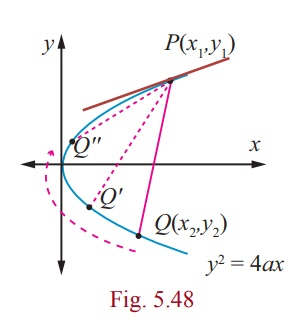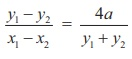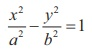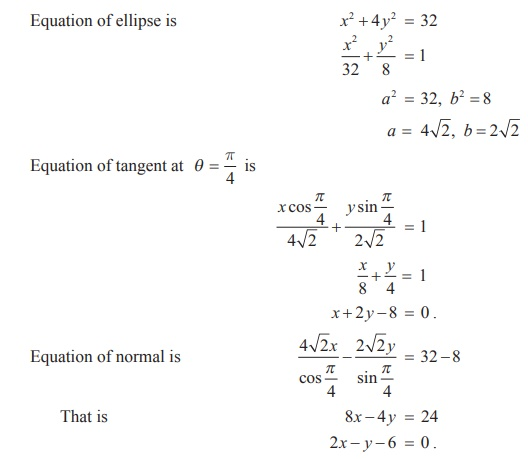Home | | Maths 12th Std | Tangents and Normals to Conics

# Tangents and Normals to Conics

Tangent to a plane curve is a straight line touching the curve at exactly one point and a straight line perpendicular to the tangent and passing through the point of contact is called the normal at that point.

Tangents and Normals to Conics

Tangent to a plane curve is a straight line touching the curve at exactly one point and a straight line perpendicular to the tangent and passing through the point of contact is called the normal at that point.

## (i) Equation of tangent in cartesian form

Let P ( x1 , y1 ) and Q ( x2 , y2 ) be two points on a parabola y2  = 4ax .Then, y12 = 4ax1 and y22 = 4ax2,

and y12y22 = 4a(x1 x2).

Simplifying,, the slope of the chord PQ.

Thus, represents the equation of the chord PQ.

When Q P , or y2y1 the chord becomes tangent at P.

Thus the equation of tangent at (x1 , y1) is

yy1 = 4a/2y1 (x - x1) where 2a/y1 is the slope of the tangent ... (1)

yy1y12 = 2ax - 2ax1

yy1 - 4ax1 = 2ax - 2ax1

yy1 = 2a ( x + x1)

## (ii) Equation of tangent in parametric form

Equation of tangent at (at2 , 2at) on the parabola is

y(2at) = 2a(x + at2)

yt = x + at2

## (iii) Equation of normal in cartesian form

From (1) the slope of normal isy11/2a

Therefore equation of the normal is2ay − 2ay1  = − y1x + y1x1

xy1 + 2ay = y1( x1 + 2a)

xy1 + 2ay = x1y1 + 2ay1

## (iv) Equation of normal in parametric form

Equation of the normal at (at2 , 2at) on the parabola is

x2at + 2ay = at2 (2at) + 2a(2at)

2a(xt + y) =  2a(at3 + 2at)

y + xt = at 3 + 2at

### Theorem 5.6

Three normals can be drawn to a parabola y2 = 4ax from a given point, one of which is always real.

### Proof

y2 = 4ax is the given parabola. Let (α, β) be the given point.

Equation of the normal in parametric form is

y = – tx + 2at + at3 ... (1)

If m is the slope of the normal then m = −t .

Therefore the equation (1) becomes y = mx − 2am am3.

Let it passes through (α, β) , then

β  = ma − 2am am3

am3 + (2a α)m + β = 0

which being a cubic equation in m , has three values of m . Consequently three normals, in general, can be drawn from a point to the parabola, since complex roots of real equation, always occur in conjugate pairs and (1) being an odd degree equation, it has atleast one real root. Hence atleast one normal to the parabola is real.

## 2. Equations of tangent and normal to Ellipse and Hyperbola (the proof of the following are left to the reader)

(1) Equation of the tangent to the ellipse(2) Equation of the normal to the ellipse(3) Equation of the tangent to the hyperbola(4) Equation of the normal to the hyperbola## 3. Condition for the line y = mx + c to be a tangent to the conic sections

### (i) parabola y2= 4ax

Let (x1 , y1 ) be the point on the parabola y2 = 4ax . Then y12 = 4ax1      … (1)

Let y = mx + c be the tangent to the parabola .. (2)

Equation of tangent at ( x1 , y1 ) to the parabola from 5.6.1 is yy1  = 2a ( x + x1 ) …. (3)

Since (2) and (3) represent the same line, coefficients are proportional.So the point of contact is ( a/m2 , 2a/m ) and the equation of tangent to parabola is y = mx + a/m .

The condition for the line y = mx + c to be tangent to the ellipse or hyperbola can be derived as follows in the same way as in the case of parabola.

### (ii) ellipseCondition for line y = mx + c to be the tangent to the ellipseis c2 = a2m2 + b, with the point of contact isand the equation of tangent is y = mx  ± √[a2m2+b2] =.

(iii) HyperbolaCondition for line y = mx + c to be the tangent to the hyperbolais c2 = a2m2 – b2, with the point of contact isand the equation of tangent is y = mx  ± √[a2m2 - b2] =.

### Note

(1) In y = mx  ±, either y = mx +or y = mx –is the equation to the tangent of ellipse but not both.

(2) In y = mx  ±, either y = mx +or y = mx –is the equation to the tangent of hyperbola but not both.

### Results (Proof, left to the reader)

(1) Two tangents can be drawn to (i) a parabola (ii) an ellipse and (iii) a hyperbola, from any external point on the plane.

(2) Four normals can be drawn to (i) an ellipse and (ii) a hyperbola from any external point on the plane.

(3) The locus of the point of intersection of perpendicular tangents to

(i) the parabola y2 = 4ax is x = −a (the directrix).

(ii) the ellipse( called the director circle of ellipse).

(iii) the hyperbola(called director circle of hyperbola).

### Example 5.27

Find the equations of tangent and normal to the parabola x2 + 6x + 4 y + 5 = 0 at (1, −3).

### Solution

Equation of parabola is x2 + 6x + 4 y + 5 = 0 .

x2 + 6x + 9 − 9 + 4 y + 5 = 0

(x + 3)2 = −4( y −1)

Let X = x + 3,Y = y −1

Equation (1) takes the standard form

X2 = −4Y

Equation of tangent is XX1 = −2(Y + Y1 )

At (1, −3)         X1 = 1+ 3 = 4; Y1 = −3 −1 = −4

Therefore, the equation of tangent at (1, −3) is

(x + 3)4 =  −2( y −1− 4)

2x + 6  =  − y + 5 .

2x + y +1 =  0 .

Slope of tangent at (1, −3) is −2 , so slope of normal at (1, −3) is ½

Therefore, the equation of normal at (1, −3) is given by

y + 3 = 1/2 (x−1)

2 y + 6  = x −1

x − 2 y − 7  = 0 .

### Example 5.28

Find the equations of tangent and normal to the ellipse x2 + 4 y2 = 32 when θ = π/4.

### Solution

Equation of ellipse is x2 + 4y2= 32Aliter= (4, 2)

Equation of tangent at θ = π/4 is same at ( 4, 2).

Equation of tangent in cartesian form isx + 2 y - 8 = 0

Slope of tangent is – 1/2

Slope of normal is 2

Equation of normal is y - 2 = 2(x - 4)

y - 2x + 6 = 0.

Tags : Equation, Definition, Theorem, Proof, Solved Example Problems , 12th Mathematics : UNIT 5 : Two Dimensional Analytical Geometry II
Study Material, Lecturing Notes, Assignment, Reference, Wiki description explanation, brief detail
12th Mathematics : UNIT 5 : Two Dimensional Analytical Geometry II : Tangents and Normals to Conics | Equation, Definition, Theorem, Proof, Solved Example Problems